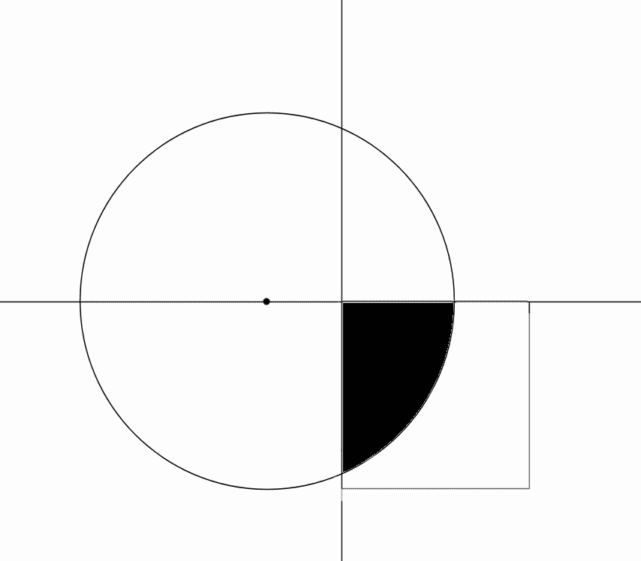# Equal Areas In Equal Time

Calculus Level 3A square $5 \times 5$ is positioned in the $4^\text{th}$ quadrant intersecting part of a circle of radius $5$ and centered at $(-2, 0)$. The square is rotated counter-clockwise about the origin.

Through what angle (in degrees) does the square have to be rotated until $\frac 1 2$ of its area intersects with the circle?

[Answer to nearest 3 decimal places]

×